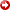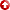[First Hit]Spatial Mechanics of Biostratigraphic Gap Formation

Michael L. McKinney

A quantitative model describes how biostratigraphic gaps form. Specifically, I show that three aspects of fossils determine the average biostratigraphic gap size (i.e., average vertical distance between adjacent fossils). These aspects are the size, shape, and number of fossils in the rock volume. The larger, more spherical, and more numerous the fossils are, the less the average gap is between them. Stereological principles show that larger, spherically shaped, and more numerous particles in a given volume have a greater chance of being intersected by a random plane (i.e., cross section) and, hence, of being exposed, which reduces the average gap size. Based on theory and rigorous mathematical modeling, I show that this relationship may be expressed as G = 0.354/^radical(Nkx), where: G is average gap size; N is number of fossils/unit volume; k is a fossil shape factor; and x is mean fossil length. Thus, the inverse relationship between gap size and these factors isnonlinear. An important implication is that small, irregularly shaped fossils must occur in much greater abundance than large, spherical forms to achieve the same level of biostratigraphic resolution. For instance, the model indicates that it takes about 20 times as many 1-cm long rod-shaped fossils to achieve the same average gap size as 10-cm long spherical forms. Therefore, where the two forms occur in similar abundances, the smaller, irregular forms ould have an average biostratigraphic gap about 450% greater than the larger, spherical forms.

AAPG Search and Discovery Article #91043©1986 AAPG Annual Convention, Atlanta, Georgia, June 15-18, 1986.